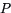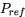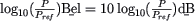Deutsche Versionprefixes | length | area | volume | weight | pressure | temperature | time | energy | power | density| velocity | acceleration | force

Conversion of dB (decibel) to bel
Logarithmic  ratios as levels (prefixes)

Conversion of international level units decibels and bels
 kilobels (kB) hectobels (hB) decabels (daB) ● bels (B)
 decibels (dB) centibels (cB) millibels (mB) microbels (µB)
 The level L is the ratio we are using with sound recording techniques, that is in bel (after Alexander Graham Bell) or in tenth part of it as decibel. Definition: dB is the twentyfold decadic logarithm of the ratio of two linear values x1 and x0: Level L = 20 × log (x1 / x0), where x0 is the reference point. Question: The dynamic region of a standard audio CD is 96 dB. How many bels are this?

 Help: 1 dm = 0.1 m and 1 dB = 0.1 bel  1 m = 10 dm and 1 bel = 10 dB

A given power level, measured with respect to the reference power level,
would be equal to. So, 1 bel = 10 dB.

Conversion from neper to decibel and vice versa

 Note: There is no conversion formula for measured                   dB values to weighted dBA values or vice versa.